## VB Universe3D - Universe 3D Project support

### Introduction and Purpose

Project "VB Universe3D" is to perform a simulation of a Universe filled with Black Holes. Project "VB Universe3D" is Test 3 of program Sagittarius A* Project - Test 3
The Black holes are situated at equal spaced distances throughout the whole universe.
Each Black hole is accompanied with a small BH which circulates around the large BH.

The Universe 3D Project is part of two other projects.

• The planet 3D project which is used to simulate the planets around the Sun. Part of this simulation is also used to study the influence of the movement of the Sun through our Galaxy, specific in relation to the planet Mercury. For more detail select this link: VB Planet3d Project
• The Sagittarius 3D project which is used to simulate 10 mini BH's around the Black Hole in the center of our Galaxy i.e. Sagittarius A*.
For more detail select this link: Sagittarius A* Project
• The Universe 3D project which is the subject of this document.
It should be mentioned that The Universe 3D project does not really involves any simulation. It is much more a discussion document of what is involved to perform a simulation in which different BH's are involved. Specific to compare this with Newton's Law versus SR and GR.

### Program Description

The program consists of 2 Forms: Control, Display
1. The Control Form is used to select a Test. There are 3 tests or cases. Each results in a drawing. Certain drawings require additional parameters.
2. The Display Form shows the drawing of each Test.

### "VB DRaw Sagittarius" Form - OperationDisplay 1 The "VB Draw Sagittarius control" form is used to select and perform a simulation. First select a particular Test. Select Test 3. Next Select Sub Test. Select Sub Test 1 Next Select Start Next the simulation will show the Displays 2 to 6 as explained below.

### Display Form - Test 3 - Operation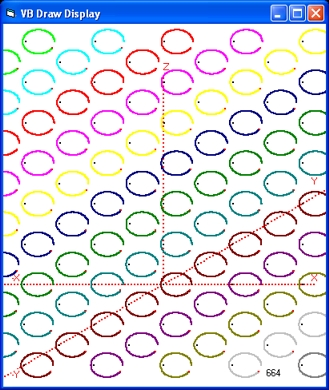Display 5 Display 5 shows an image of the 3D universe with Black Holes at equally spaced distances. Each BH is accompanied with one star or mini BH To observe the 3D world it is important to imagine that each Black Hole is observed many times in different parallel planes. The first plane is the X,0,Z plane. The origin of this plane is the point (0,0,0) The second plane is the X,1,Z plane. The origin of this plane is the point (0,1,0). This is a point in the direction of the point (0,0,0) at 2 o'clock. These seem the same black holes as the black holes in the first plane but they are not. The third plane is the X,2,Z plane. The origin of this plane is the point (0,2,0). In total there are 9 planes along the Y axis, starting from the point (0,-4,0) and finishing at the point (0,4,0) All these planes are parallel to the X and Z axis. We can also study planes parallel to the Y and Z axis. The first plane is the 0,Y,Z plane. The origin of this plane is the point (0,0,0) The second plane is the 1,Y,Z plane. The origin of this plane is the point (1,0,0). This is a point in the direction of the point (0,0,0) at 3 o'clock. These seem the same black holes as the black holes in the first plane but they are not. The third plane is the 2,Y,Z plane. The origin of this plane is the point (2,0,0). More planes can be identified i.e. through the points -1,Y,Z and -2,Y,Z We can also study planes parallel to X and Y axis. These are all horizontal.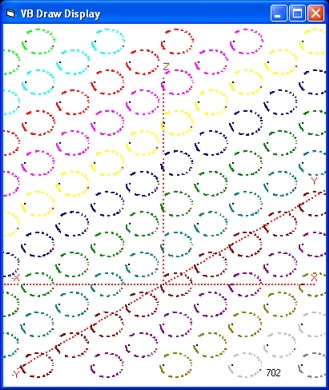Display 6 Display 5 shows an image of the 3D universe with Black Holes at equally spaced distances throughout the total universe of which only a 'small' part is visible. Each BH is accompanied with a revolving star. The star is simulated using Newton's Law as a simple binary system. One revolution requires roughly the calculation of 775 positions. In Display 5 all these positions are shown, which give the impression of a continuous line. Display 6 shows the same simulation and each revolution requires also 775 calculations but not all the positions are visible. In fact only 50% are shown in a distinctive colour. All the others are shown in white. What is important the positions are shown in groups. Group #1 of 25 positions is shown in red. Group #2 of 25 points in white. Group #3 in red and the same with the groups 5,7,9,11 until group 31. This completes one revolution. (31*25=775). The next revolution starts a white group and now all the even groups are in red. The result is that you can follow where the star is. What is more important you can follow the evolution of each star instantaneous throughout the total universe. To get an idea of how this works, please select Display 6. When selected the display switches instantaneous to a different world view i.e. from calculation 702 into 2000. In fact you get an instantaneous view of the state of the total universe independent of the observer. This is an important concept, which requires a deeper investigation. An observer observes with his eyes and what he or she observes is in the past. In Display 5 and 6 this time delay is not included. Both displays show no horizon. One virtual experiment you can perform is to connect a clock to each black hole a clock and observe that all clocks show the same count. You can also connect a clock to each star and observe that all these moving clocks show less counts than the Black Holes.
• You can also perform the following experiment. Take a (rigid) rod which has the same length as the distance between two BH's. For example this rod makes a connection between the BH's (0,0,0) and (0,1,0) along the Y axis. Next move this rod.
Now we get the critical question: Is there any length contraction involved? And if yes what is the explanation. Specific requires this explanation light (photons) or the speed of light.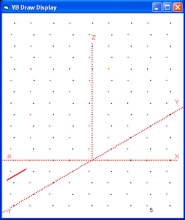Display 7A - 5Display 7B - 500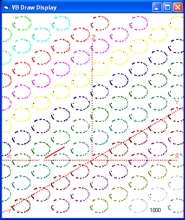Display 7C - 1000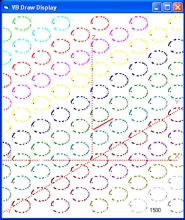Display 7D - 1500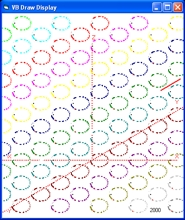Display 7E - 2000
The above 5 displays (7A-7E) show the behaviour of a rod at equal count increments of 500 counts. The distance between the position of the rod between two pictures increases which implies an increasing speed. The length of the rod in each picture is the same.
• The first question to answer is if that is in accordance with physical observations (measurements). That is difficult to answer.
• The second question to answer is why not?
• And if not what is the physical reason that causes any length contraction.
• Similar questions can be raised considering the behaviour of a moving clock which internally uses light signals. Such a clock will run slower because the light path is longer compared with a clock at rest. A clock connected with a BH will run faster compared with a clock connected to a star. In fact all the clocks, each connected to a different star in the Sagittarius Project will run at a different slower rate as of the imaginary clock part of the BH.
• When you connect an engine to the front of the clock the engine will stretch the rod and become longer. But this has nothing to do with photons. When you connect an engine at the back, this engine will push the rod and the rod will become shorter. Again this behaviour has nothing to do with photons. In fact all forms of length contraction are all related to the composition of the rod and or if the mass of the rod is constant.

### Reflection 1 - 3D universe

The purpose of Displays 5 and 6 is to show how the universe looks from the physical perspective. Specific assuming that there are only BH's i.e. invisible objects. In this world there are no photons. This implies that a physical process, like how stars or BH's rotate around a BH, cannot be explained using photons or the speed of light. The only way is to use gravitons and or the speed of gravitons.
The physical issue is that gravitons behave very difficult as photons, in the sense that if a photon has to go from A to B and there is an object in between the object has to go around this object which we observe as a gravitational ring. In the case of a graviton, the graviton will travel in a straight line through the object, as if it does not exist. In that case the mathematics which describes this path is much simpler.

This problem is even much more complex: In order to observe, we use our eyes, which in turn use photons. At the same time almost all what we observe, specific the physical behaviour of stars and the planets, does not require photons and the speed of light. The same is for the physical laws that describe this behaviour. Along that same line all objects in the universe influences each other. This requires one common approach and one reference frame and invalidates the use of different reference frames i.e. one for each galaxy.

The idea behind Display 5 and 6 is to study the universe from the point of view of all objects involved and not from the point of view of a single observer. The fact that the universe is everywhere the same is mainly to show that there is nothing special and that the rules (laws) that apply are valid everywhere. Each 'galaxy' in Display 5 and 6 is identical and consists only of one star. The result of a simulation, of one BH and one star, shows that such a binary system is very stable. The physical assumption is that there exists no mass transport between both objects. The question is, if in case of star S62 this assumption is realistic because of the high speeds involved when S62 is at perihelion. Physical it is possible that S62 type stars can loose mass. This same question arises if all stars considered are like S62.

### Reflection 2 - Time dilation versus Clock dilation

In the above text Length Contraction is discussed. The general lesson within the SR context is, assuming that nothing visible is observed based on human observations, that length contraction physical does not exist. All changes in length, observed by humans, are illusions. That does not mean that moving objects cannot loose material or mass and that they decrease in size or weight, but all these changes are permanent.

Time dilation as the name suggest, implies that time is not constant. To understand that you must first have a clear or accepted definition what time is. That is not simple. We humans experience (are aware) of our existence and we experience that always or all the time. We humans also experience that there exists an external world outside us. We call that the universe. We also assume that these objects existed before we were born and will exist after we die. As such based on our experience we can claim that the universe exists in time, over a long period and we humans exist in time, over a short period. Time is not something physical compared with the existence and the evolution of the universe. As such we cannot claim that time changes because if it does we have to explain: changes compared to what, and that is impossible. Based on our experience we can claim that the universe physical changes compared what we have observed yesterday but the same cannot be said about time. As such there exists nothing, which we can define as Time dilation
Clock dilation is something physical. Clock dilation is related to the physical behaviour of a clock, specific to the behaviour of different moving clocks. This type of physical behaviour is already known for a long time, specific related for clocks on board moving ships. Time keeping for ships is important to calculate their positions. The general idea behind a clock is to have something which behaves in a cyclic (or oscillating) pattern and each cyclic is considered a count. In order to measure time with a clock, the clock has to be calibrated. Roughly speaking that means that at noon you must reset your clock and measure at noon the next day, the number of counts of your clock. When that number is n, a simple calculation shows (roughly) that 1 count = 24*60*60/n seconds.
The main assumption is that the clock should not move, because the physical path that the oscillating object follows will become longer. For a clock using light signals this is (simple?) to explain. Such a clock uses a light signal which bounces (up and down) between two mirrors. When a clock is at rest this is a vertical straight path up and down. When the clock moves towards the right the mirrors also move towards the right. The result is that the path that the reflected light signal follows becomes longer as original and as such the clock ticks less.
The main problem is exactly what means at rest. However the main underlying cause of Clock dilation that the clock which travels the longest path through space, ticks the slowest.

The underlying lesson is that performing physics, measuring time, don't use moving clocks.

### Reflection 3 - Gravitational mass versus inertial mass and uncertainty principle.

The first question to answer is: how do you measure mass.
There are two possible ways: (1) By means of volume. (2) By means of mutual attraction i.e. gravitational.
• The amount of matter can only be measured by means of volume if the material (or composition) involved is the same. That means both objects are 100% gold or both 50% gold and 50% iron. etc.
To measure the amount you put an object in a bucket filled with water. At the same time you take care that all the water that goes over the rim flows into a measuring-glass. The height of the measuring-glass is a measure of the volume of the object. Because the composition of both objects is the same the height is also a measure of their mass. That means m2 = (h2/h1) * m1
• The amount of mass of two objects can also be measured based on their mutual their attraction. The easiest way is with two objects, which revolve around each other like a binary system, in a by approximation empty universe. In that case when the mass of the two objects are identical it is possible that the circular path through space is identical i.e. the radius r1 and r2 around the center of gravity of each objects is the same, to be established by an outside observer.
When this is not the case the radius r1 of object m1 and the radius r2 of object m2 are related to each other using the following equation: r1*m1 = r2*m2 with r1 being the distance with the center of gravitation. Again as before to be established by an outside 'observer'

The uncertainty principle expresses the fact that we humans can only perform measurements within certain limits. The universe evolves and changes in time. There are two specific reasons why uncertainty principle is mentioned:

• The first reason is that the uncertainty principle in no way has anything to do how the 3D universe evolves in time.
• The second reason is because we humans can not study at all levels the details of how these changes are physical interrelated. Specific at quantum level.
For more information: Is the 'uncertainty principle' a law of physics? Discussion in Sci.Physics.Research as of 18 March 2017.

Now we return back to the 3D universe in total and consider the question: what are the rules that describe how objects (stars) interact which each other. Part of the answer lies in the observation that all what interacts is mass or gravitational mass. That means all black holes, all visible matter and all dark matter. There exists no difference between each.

### Feedback

None

Created: 3 September 2020